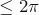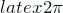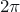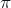# Every convex polyhedron can be refolded to a different one

Choose your favorite convex polyhedron in the space. Make sure it is convex, since the current post is restricted to that kind of polyhedra

An unfolding of your convex polyhedron is a development of its surface to a single polygon in the plane (possibly overlapping). In order to obtain an unfolding, the surface of the polyhedron must be cut along a spanning tree of its vertices. If the spanning tree uses only edges of the polyhedron (i.e., only cuts along the edges are allowed) you have an edge unfolding.

Conversely, a folding of a polygon in the plane (not necessarily coming from unfolding a polyhedron) is defined as an identification of the boundary points of the polygon, in such a way that the three conditions of Alexandrov’s theorem are satisfied: (1) The identifications (or “gluings”) close up the perimeter of the polygon, without gaps or overlaps; (2) The resulting surface is homeomorphic to a sphere; and (3) Identifications result in ansurface angle glued at every point. If these conditions are fulfilled, Alexandrov’s theorem guarantees that the folding produces a convex polyhedron, unique once the gluing is specified 1. An important observation: It is not required that whole edges are identified to whole edges.

It is well known that the cube can be edge-unfolded to a Latin cross (which is just one of its 11 edge unfoldings), but it is not so well known that this Latin cross can also be refolded to a polyhedron different from the cube. For instance, it can be refolded to an octahedron,

to a tetrahedron, or to a pentahedron2 (the companion videoMetamorphosis of the cube is strongly recommended). Actually, this Latin cross can be refolded to 22 distinct convex polyhedra different from the cube.

Thus, one can wonder:

Does every convex polyhedron have an edge unfolding which can be refolded to a different convex polyhedron?

The answer is no. In a recent paper, Demaine et al.3 define edge-refold rigid convex polyhedra as those for which every edge unfolding can only refold back to the original polyhedron. They prove that the dodecahedron is edge-refold rigid, since any of its 43,380 edge unfoldings can only be refold back to the dodecahedron. The authors also prove that 11 of the 13 Archimedean solids are edge-refold rigid.

The tool Demaine et al. use to prove these results is a (surprisingly simple) sufficient condition for a polyhedron to be edge-refold rigid: The smallest angle among its faces being greater than the largest curvature among its vertices. This vertex curvature is defined as the “angle gap” at the vertex, i.e.,minus the sum of the incident angles for all the incident faces.

Furthermore, the authors show that any polyhedron fulfilling this condition admits a slight perturbation of its vertices leading to an uncountable number of edge-refold rigid polyhedra.

On the contrary, the convex polyhedra which are not edge-refold rigid are defined to be edge-refold transformers. Apart from the cube, the truncated tetrahedron, the icosahedron and the cuboctahedron are shown to be edge-refold transformers. Furthermore, the authors establish that there is an infinite class of edge-refold transformers by proving that, for every tetrahedron, at least one of its 16 edge unfoldings can be refolded to an incongruent polyhedron.

Recall that until now only edge unfoldings have been considered, allowing only cuts along the edges of the polyhedron. What happens if we also allow cutting through faces?

In such a more general setting, the paper by Demaine et al. proves the following surprising result:

Every convex polyhedron may be unfolded to a polygon in the plane, and then refolded to a different convex polyhedron, actually with a larger number of vertices.

In other words, in the general setting no convex polyhedron is refold-rigid. The proof is split into two cases using a Lemma which states that, for every convex polyhedron, either there is a pair of vertices whose sum of vertex curvatures is greater thanor there is a pair of vertices having each a vertex curvature not greater than.

The paper concludes with several interesting and intriguing open questions, among which the following one seems specially appealing:

Which refolding of any of the 11 edge unfoldings of the cube achieves the maximum volume? In particular, is it the cube itself?

## References

1. Erik D. Demaine and Joseph O’Rourke. Geometric Folding Algorithms: Linkages, Origami, Polyhedra. Cambridge University Press, July 2007.
2. Erik D. Demaine, Martin L. Demaine, Anna Lubiw, Joseph O’Rourke, and Irena Pashchenko. Metamorphosis of the Cube. 8th Annual Video Review of Computational Geometry, associated with the 15th Annual ACM Symposium on Computational Geometry (SoCG’99).
3. Demaine E.D., Demaine M.L., Itoh J.I., Lubiw A., Nara C. & OʼRourke J. (2013). Refold rigidity of convex polyhedra, Computational Geometry, 46 (8) 979-989. DOI: 10.1016/j.comgeo.2013.05.002